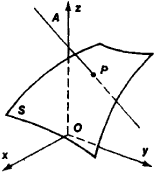# Normal Derivative

## normal derivative

[′nȯr·məl di′riv·əd·iv]
(mathematics)
The directional derivative of a function at a point on a given curve or surface in the direction of the normal to the curve or surface.
McGraw-Hill Dictionary of Scientific & Technical Terms, 6E, Copyright © 2003 by The McGraw-Hill Companies, Inc.
The following article is from The Great Soviet Encyclopedia (1979). It might be outdated or ideologically biased.

## Normal Derivative

of a function defined in space (or in a plane), the derivative in the direction of the normal to some surface (or to a curve lying in the plane). Let S be a surface, P a point on S, and f a function in some neighborhood of P. Then the normal derivative of f at P is equal to the limit of the ratio of the difference f(A) — f(P) over the distance from A to P, where A is a point on the normal to S at P that approaches P from one side of S (see Figure 1).Figure 1

We distinguish between the derivative of f with respect to the outward-drawn and the inward-drawn normals to S, depending on the direction from which A approaches P. Consideration of normal derivatives is particularly important in the theory of boundary value problems.

References in periodicals archive ?
Here [[xi].sub.0] = ([[tau].sub.0],[[psi].sub.0]) is a fixed source point, [xi] = (r, [psi]) is integration variable and [q.sub.1]([xi]) = [partial derivative][u.sub.1]/[partial derivative]r is normal derivative of the potential function on [[psi].sub.1].
If we further demand the normal derivative to be constant on S, then we arrive at probably the most common boundary condition used in developing a Neumann-Green's function :
The current flowing into the medium is expressed in terms of the normal derivative of the potential, taken from the side of the medium.
Here [DELTA] is the Laplacian in [R.sup.m], [ohm] [subset] [R.sup.m] is a bounded domain with smooth boundary [partial derivative][ohm] and [partial derivative]/[partial derivative]n is the outward normal derivative to [partial derivative][ohm].
Here the outward normal derivative is [mathematical expression not reproducible]; then
where R(P,Q) is the distance between field point P (x, y, z, t) and boundary point Q ([x.sub.0], [y.sub.0], z), [partial derivative]/[partial derivative][n.sub.Q] is normal derivative to S at point Q.
n is the unit outward normal field along the boundary [GAMMA], D is the Levi Civita connection on M and [partial derivative]y/[partial derivative]n = [<Dy, n>.sub.g] is the normal derivative.
The normal derivative [partial derivative]u/[partial derivative]n on S is calculated in an analogous way and, thus, the residual [PSI] of the boundary condition on S is updated.
Note that [partial derivative][OMEGA] is part of the interface because the boundary condition for the normal derivative is only enforced weakly through the penalty term in (1.3).
At the outlet, the uniform axial velocity was used to ensure continuity, and tangential velocities were computed from a zero normal derivative condition.
The local potential V(M[member of][OMEGA]) can be written using Green's theorem  in terms of V(P) and of the normal derivative [partial derivative]V(P)/[partial derivative]n with P being any point on the boundary S (with no overhangs) of [OMEGA]:

Site: Follow: Share:
Open / Close